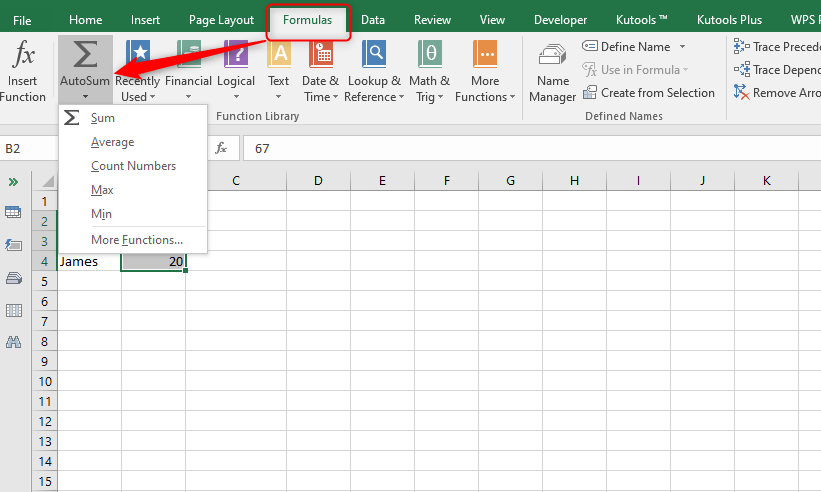# How to find the highest number in a column

Finding the highest number in Excel can be very useful when you have a long list of items. But some people do not know how easily one can find the highest number. Others find the highest number manually making it a very tiresome process. Although that can be an effective way for very small filled columns, you will struggle with a larger list.

## 1. Using a Formula

The formula for finding the highest number in a column is =Max ().

Steps

Enter the formula =Max () in an empty cell.Select the column that you want to find the highest value using the mouse. But you can also enter the values manually to select the cells. Check the images belowHit enter and the highest number will be populated in the empty cell that you selected.To find the lowest number in the same column then you will have to use a different formula but follow the same procedure for the highest number. The formula for finding the lowest number is =Min(). Then enter your column values inside the parenthesis and hit enter. Also, you can do this by highlighting the cells using the mouse.

## 2. Using Auto sum method

The second method for finding the highest number in a column is through the Auto sum.
Steps
1. Highlight the cells2. Click on the Formulas tab

3. Then select Autosum4. You will get a few options on the drop-down menu.

NB. You should make sure to leave a blank cell below the highlighted cell. This is where the highest number of the column will appear. If you want to find out the lowest number of the column repeat the same process but choose Min instead of Max from the drop-down menu

## 3. Finding Max value in a group

When you want to find the max value for a group of columns follow these steps.

1. Type max formula in an empty cell

2. Type the opening parenthesis3. Hold down the control key and start highlighting the ranges and cells in the sheet4. After you are done highlighting, release the control button and hit enter. Your max value will be populated automatically

## 4. How to get the max date in an excel column

Sometimes you may want to find the highest date in an excel worksheet. The best thing is that the excel system treats the dates just like serial numbers. This means that the MAX function will handle them just like the normal numbers. For example, if you want to find the latest payday in C3: C8 just use the normal MAX formula. Since the date is treated as a normal number, you can use all the above techniques when it comes to finding the highest number.